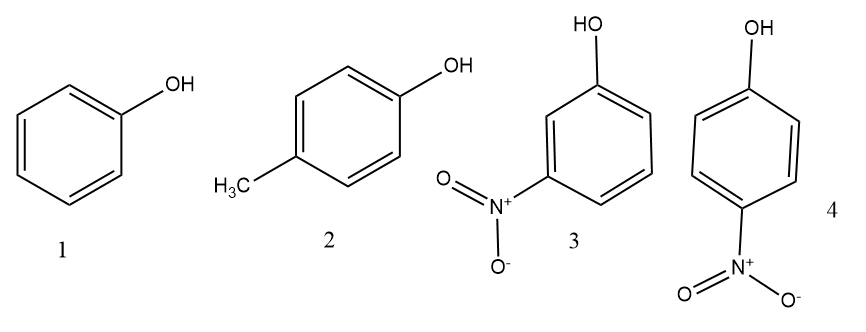# In the following compounds. The decreasing order of acidity is1. A

$\mathrm{I}>\mathrm{IV}>\mathrm{III}>\mathrm{II}$

2. B

$\mathrm{I}>\mathrm{IV}>\mathrm{I}>\mathrm{II}$

3. C

$\mathrm{II}>\mathrm{I}>\mathrm{III}>\mathrm{IV}$

4. D

$\mathrm{IV}>\mathrm{III}>\mathrm{I}>\mathrm{II}$

FREE Lve Classes, PDFs, Solved Questions, PYQ's, Mock Tests, Practice Tests, and Test Series!

+91

Verify OTP Code (required)

I agree to the terms and conditions and privacy policy.

### Solution:

The correct order of acidity $\mathrm{IV}>\mathrm{III}>\mathrm{I}>\mathrm{II}$.

Acidity is more, when the phenol has more number of minus I groups. Since the minus I nature will stabilise the carbanion intermediate. When we se the general order of acidity then

RCOOH > Phenol> water > alcohol > alkynes.

Ka is proportional to acidity and inversely to Pka.

## Related contentJoin Infinity Learn Regular Class Program!

Sign up & Get instant access to FREE PDF's, solved questions, Previous Year Papers, Quizzes and Puzzles!

+91

Verify OTP Code (required)

I agree to the terms and conditions and privacy policy.